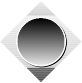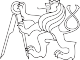# acm cz#### Czech ACM Student Chapter Czech Technical University in PragueCharles University in Prague Technical University of Ostrava Slovak University of Technology Pavol Jozef Sˇaf´arik University in Koˇsice

University of Zˇilina Masaryk University Matej Bel University in Bansk´a Bystrica University of West Bohemia

### CTU Open Contest 2015## Hacking the Screen

screen.c, screen.cpp, Screen.java, screen.py

The ZOO management had been wondering for a long time how to increase the number of children visitors to the ZOO. The solution was surprising and unexpected in many ways. They installed a huge screen past the entrance and started to display short quizzes on it. The child in the crowd who ﬁrst shouts out the answer to the quiz question is granted one day free access to the ZOO. The screen became soon very popular and various types of quizzes are routinely shown there. One type of the quiz is the math quiz containing arithmetic operations on integers. The management worries that older siblings and friends of the children might develop a math quiz screen hacking strategy: Snap the screen with the phone, run the image recognition SW which extracts formulas from the image, evaluates them, and presents the solution to the phone holder who immediately shouts out the answer.

Your task is to assess the diﬃculty of producing the screen hacking software. To get a better feel of the problem you will ﬁrst develop a simple toy model application. Your code will read the formula presented in the preprocessed form of ASCII art and evaluate it.

### Input Specification

There are multiple test cases. First line of each test case contains two integers R and C (1 ≤ R ≤ 3, 1 ≤ C ≤ 1 000). Each of the following R lines contains C characters. The whole matrix of R × C characters represents a single arithmetic formula written in ASCII art and generated by the following set of rules:FORMULA -> COMPLEX | FORMULA + COMPLEX | FORMULA - COMPLEX COMPLEX -> SQRT | FRACTION | TERM

SQRT -> \/SIMPLE

SIMPLE FRACTION -> ======

SIMPLE

SIMPLE -> TERM | SIMPLE + TERM | SIMPLE - TERM TERM -> INTEGER | INTEGER * TERM

INTEGER -> 0 | 1 | 2 | 3 | ... | 999999 | 1000000

There are also a few additional speciﬁcations regarding the layout of the formula.• The horizontal bar of each SQRT is made of one or more underscore symbols (’ ’, ascii decimal code 95) and it always occupies the uppermost line of the formula in the screen.

• When the formula occupies exactly two lines, then the ﬁrst line contains only horizontal bars of all SQRT parts of the formula.

• When the formula occupies exactly three lines, then all TERMs and all arithmetic operation symbols which are not part of any FRACTION or SQRT occupy the second line of the formula in the screen.

• The length of the horizontal bar of SQRT is the same as the length of SIMPLE under the bar.

• The fraction bar in FRACTION consists of one or more equality signs, its length is equal to the maximum of the lengths of SIMPLE above the bar and SIMPLE below the bar.

• There is always exactly one space preceding and following each arithmetic operation symbol (+, -, *) on a particular line.

• The formula exactly ﬁts in to the R × C matrix, there are no blank/empty columns in front of the whole formula or behind it.

The whole formula is evaluated according to the standard arithmetic rules. Namely: Each FORMULA and each TERM is evaluated from left to right. Each SIMPLE is also evaluated from left to right with the additional standard condition that the multiplication has higher priority than the addition/subtraction. Evaluation of SQRT and FRACTION is also standard. The value of any evaluated FORMULA, COMPLEX, SQRT, FRACTION, SIMPLE and TERM is an integer whose absolute value does not exceed 1 000 000.

There is one empty line after each test case. The input is terminated by a line with two zeros.

### Output Specification

For each test case print a separate line with the value V of the input formula.

### Sample Input

1 13

1 + 2 * 3 - 4

2 16\/3 * 4 - 3 + 10

3 5

6 * 4

===== 12

3

13

2

-3

3 13

22

3 - == - \/16

11

0 0